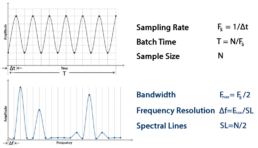Vibration measurements are performed to determine the response of machine equipment to both internal and external forces. In mechanical systems, different points of the same equipment are different in geometry, material, etc. That’s why they may have distinctive vibrations due to effects. As an example, when an electric motor operates at high frequencies, frequency of reducer output shaft will be much lower in a system consisting of electric motor and reducer in a conveyor line. Using the same parameters for vibration measurement of both parts may cause misleading measurements. In such cases, vibration measurement parameters must be edited.

In the analog-to-digital conversion (ADC), so-called sample size of the digital waveform must often be taken to reproduce the signal accurately. Vibration measurements are usually taken over a duration of time and repeated periodically. The analog signal is converted into a digital signal with samples taken at specific time intervals called sampling time (1/fs). The number of samples taken per second is called sampling rate or sampling frequency. Periodic measurement times are obtained by dividing sample size by the sampling frequency. Half of the sampling frequency (fs/2) is often referred to as Nyquist Frequency.Fig. 1 Time and Frequency Domain Parameters

Any analog signal consists of components of various frequencies. The highest frequency (fmax) component in an analog signal determines the bandwidth of that signal. Assuming that the highest frequency component for a given analog signal is fmax, according to the Nyquist-Shannon Sampling Theorem, the sampling rate should be at least 2×fmax, or twice the highest analog frequency component. It is important to understand what the sampling theorem means. If the sampling theorem is performed before sampling an analog signal, then the sampled signal exactly represents the analog signal. In this case, the sampled signal contains all the information in the analog signal. Aliasing occurs when the sampling frequency is less than 2 times the largest frequency in the analog signal (fs<2×fmax). Aliasing prevents the high frequencies in the analog signal to be seen in the spectrum and causes the signals generated by the vibration source to be misinterpreted. Just as insufficient sampling frequency causes aliasing, insufficient sampling size also weakens the frequency resolution in the spectrum and block intermediate frequencies from appearing. Before vibration measurement, not only the bandwidth and sampling frequency must be determined, but also the dynamic range of the vibration amplitude must be determined. For instance, while the measurement accuracy in the 2G range offered by Sensemore products is sufficient for low amplitude vibrations caused by bearing wear, measurements should be made with 8G / 16G intervals in high amplitude vibrations caused by a press line. Since the vibrations in the press line are low frequency and high amplitude, if the dynamic range of 2G is selected, the amplitudes that are out of range cannot be processed. If large dynamic ranges such as 16G are selected for bearing vibration measurements, the sensitivity of the sensor and the resolution of the graphics in the time/frequency domains decreases.Fig. 2 The Effect of Sampling Rate on Spectrum Bandwidth

### Aliasing

If we do not follow the sampling theorem, a phenomenon called aliasing is going to occur. In case of aliasing, analog signals are transformed to digitals signals in a different way and this will prevent high frequencies to be appear in spectrum. Therefore, aliasing is an undesirable phenomenon in which digital signals are processed. All data collectors/analyzers have built-in sampling rates to avoid aliasing. Theoretically, there should be no vibrations with a frequency more than half of this sampling rate. However, this can never be achieved in practice. That is why all analyzers have anti-aliasing filters. These are low-pass electronic filters that allow lower frequencies to pass but block higher ones. Filters eliminate all vibrations in an analog signal with frequencies greater than half the sampling rate. These filters automatically adjust to the appropriate values as the sampling frequency changes. This happens when the analyzer’s frequency range is changed by the user.Fig. 3 Aliasing

### Discrete Representation of Analog Signals

The limited high-speed sampling rate used in most measurement systems designed for vibration signals can affect the accuracy of time signals when generated. In the figure below, a signal is marked with 2.56 times lower sampling frequency and 20 times oversampling. It is clear that the signal with low sampling frequency does not correctly identify the analog signal from a careful study of the figure. The signal seems to bounce between impossible sampling points. Hovewer, it is important to understand that with the 2.56 times lower sampling frequency, the signal still contains all the information in the analog signal. This means that a spectrum calculated from the signal will be correct. The low sampling frequency only limits the time domain representation of the signal. There is no such limitation in the frequency domain. If the equipment being measured is operating at low frequency, choosing a sampling rate at very high frequencies will slow down the data flow and require more storage space. On the other hand, if the dynamic range is not selected correctly, the problem arises that the vibration peak values ​​cannot be captured even with high resolution. To avoid such problems, it is necessary to predict the dynamic range(amplitude) and bandwidth of the equipment to be measured before the measurement is made.References

• C. Scheffer, P. GirdharMachinery Vibration Analysis & Predictive Maintenance(Oxford:Elsevier, 2004)
• A. Brandt, Noise and Vibration Analysis(New Delhi: Wiley, 2011)
• Bertoletti, 2020, Nyquist-Shannon Sampling Theorem